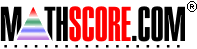Math Practice Online > free > lessons > Florida > 6th grade > Divisibility Rules

## Divisibility Rules

Find which numbers evenly divide another number.

 Sample Problems for Divisibility Rules Lesson for Divisibility Rules

### This topic aligns to the following state standards

Grade 4: Num 3. Knows rules of divisibility for 2, 3, 5, 9, and 10.
Grade 5: Num 6. Identifies and applies rules of divisibility for 2, 3, 4, 5, 6, 9, and 10.
Grade 6: Num 4. Uses divisibility rules.
Grade 7: Num 5. Applies number theory concepts, including divisibility rules, to solve real-world or mathematical problems.
Grade 8: Num 3. Applies number theory concepts, including divisibility rules, to solve real-world or mathematical problems.

Copyright Accurate Learning Systems Corporation 2008.